## Big Lump 'O' Clay

Author(s): Craig Didden & Jim Schwagle
Discrepant Event - Teacher's Guide
SED 695B; Fall 2005

-In this activity students are asked the question does the size of an object affect its density? I use clay because it is easily manipulated, and the students can make all different sizes of balls to test their hypothesis. Make sure that you go over the formula for finding the density of an object, Density=Mass/Volume. Also it is important that students understand how to find the volume of an irregular shaped object.

Principles ilustrated

• Density does not change with size.
• Finding mass and volume of an object.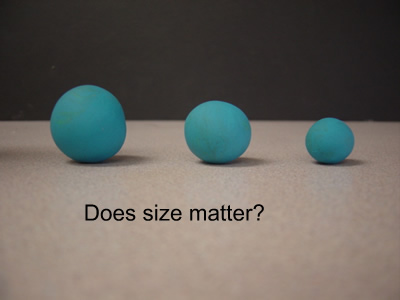Standards

Density and Buoyancy

• a. Students know density is mass per unit volume.
• b. Students know how to calculate the density of substances (regular and irregular solids and liquids) from measurements of mass and volume.

Handouts/Worksheets

Big Lump'O' Clay Write-Up

Density Questions

Density Worksheet

Questioning Script

Prior knowledge & experience:

• How to use a triple beam balance
• How to read a graduated cylinder
• Density formula D=M/V
• Water displacement method

Root question:

• Will the density of an object change if you change the size of that object?
• How do you determine the density of an object

Target response:

Common Misconceptions:

• That if you change the size of an object it changes the density.
• That the object has to be broken into identical halves for the densities to be the same.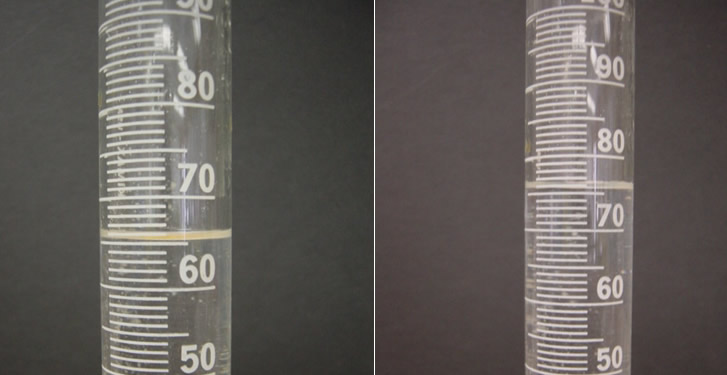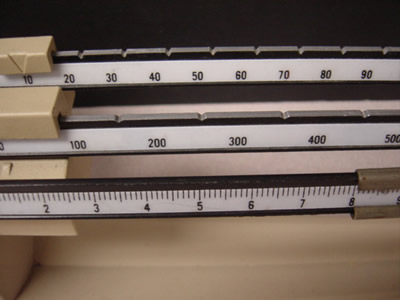These are the results of measuring the mass and volume of one half of the clay ball.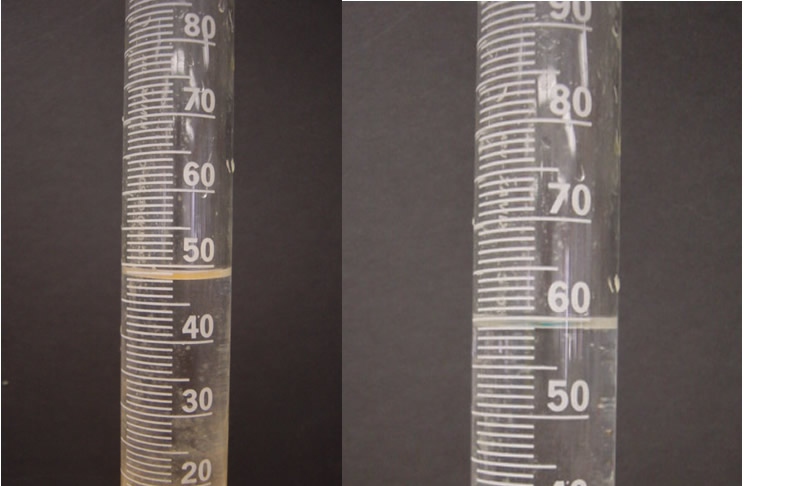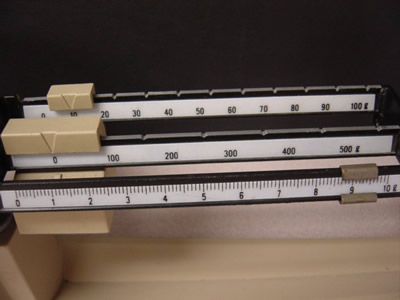These are teh results of measuring the other half of the clay ball.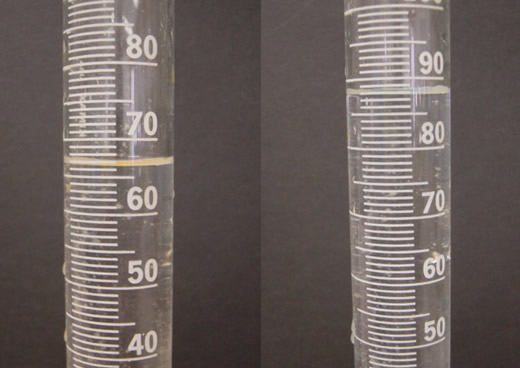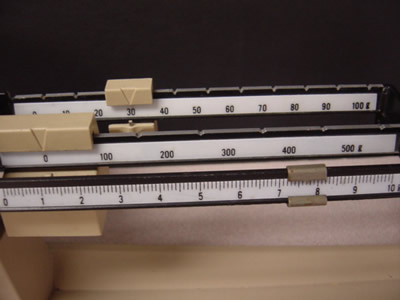These are the results of measuring th ewhole ball of clay.

Study Questions

1. How do you determine the volume of an irregular shaped object?

2. How do you determine the volume of an object with equal sides?

3. What is the equation for detemining the density of an object?

4. Explain the water displacement method of finding density.

5. What are some problems with using a triple beam balance to determine the mass of an object?

6.